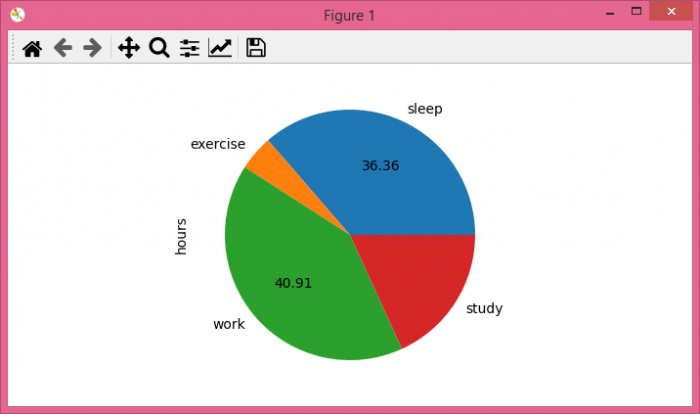# Conditional removal of labels in Matplotlib pie chart

To remove labels from a Matplotlib pie chart based on a condition, we can take the following steps −

• Set the figure size and adjust the padding between and around the subplots.
• Create a Pandas dataframe of wwo-dimensional, size-mutable, potentially heterogeneous tabular data.
• Plot a pie chart, using pie() method with conditional removal of labels, such that if %age value is greater than 25, then only keep labels, otherwise remove them.
• To display the figure, use show() method.

## Example

import pandas as pd
from matplotlib import pyplot as plt

# Set the figure size
plt.rcParams["figure.figsize"] = [7.00, 3.50]
plt.rcParams["figure.autolayout"] = True

# Create a Pandas dataframe
df = pd.DataFrame(
{
'activities': ['sleep', 'exercise', 'work', 'study'],
'hours': [8, 1, 9, 4]
}
)

# Pie chart with conditional removal of labels
df.set_index('activities').plot.pie(y='hours', legend=False, autopct=lambda p: format(p, '.2f') if p > 25 else None)

plt.show()

## Output

It will produce the following outputNotice that the pie chart shows the labels only when the percentage of value is greater than 25 (as per the condition). Since the values of "exercise" and "study" are less then 25, the pie chart doesn't reflect those labels.